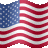# Offset Regression Bands Indicator and Strategy for ThinkorSwim#### BenTen

Staff
VIP
This strategy was based on the Regression Channel indicator for ThinkorSwim. According to the original page, the strategy is based on offset regression bands and works well with indices and diverse ETFs.

I tested it on several large cap stocks and the signals worked out well most of the time too.

Some examples include AMD, MCD, and SPY. There is a built-in watchlist in ThinkorSwim called Indices and All ETFs. You can quickly pull them up and test out the tickers on there.### thinkScript Code for Long

Rich (BB code):
``````# stdrev buy
# Original https://github.com/dougives/stdrev/blob/master/stdrev.thinkscript

def OPEN_ACTUAL_TIME = 0930;
def CLOSE_ACTUAL_TIME = 1600;
def is_trading_hours = If((SecondsFromTime(OPEN_ACTUAL_TIME) >= 0 && SecondsTillTime(CLOSE_ACTUAL_TIME) > 0), 1, 0);

def stoploss = close < close[-10] * 0.95;

def rsi = RSI("average type" = "EXPONENTIAL");

input price = close;
input deviations = 1.1;
input short_gap = 0.3;
input full_range = no;
input length = 1024;
input overshoot = 0.2;
input only_when_open = no;

def regression;
def std_deviation;
if (full_range) {
regression = InertiaAll(price);
std_deviation = StDevAll(price);
} else {
regression = InertiaAll(price, length);
std_deviation = StDevAll(price, length);
}

def upper = regression + deviations * std_deviation;
def breaker = regression + overshoot * std_deviation;
def middle = regression;
def lower = regression - deviations * std_deviation;

def upper_s = regression + (deviations + short_gap) * std_deviation;
def breaker_s = regression - overshoot * std_deviation;
def middle_s = regression;
def lower_s = regression - (deviations + short_gap) * std_deviation;

def sell2c = price crosses above breaker;

AddOrder(OrderType.SELL_TO_CLOSE, condition = sell2c, price = close,100, tickcolor = Color.GREEN, arrowcolor = Color.GREEN, name = "Cover");``````

### thinkScript Code for Short

Rich (BB code):
``````# stdrev sell
# Original https://github.com/dougives/stdrev/blob/master/stdrev.thinkscript

def OPEN_ACTUAL_TIME = 0930;
def CLOSE_ACTUAL_TIME = 1600;
def is_trading_hours = If((SecondsFromTime(OPEN_ACTUAL_TIME) >= 0 && SecondsTillTime(CLOSE_ACTUAL_TIME) > 0), 1, 0);

def stoploss = close < close[-10] * 0.95;

def rsi = RSI("average type" = "EXPONENTIAL");

input price = close;
input deviations = 1.1;
input short_gap = 0.3;
input full_range = no;
input length = 1024;
input overshoot = 0.2;
input only_when_open = no;

def regression;
def std_deviation;
if (full_range) {
regression = InertiaAll(price);
std_deviation = StDevAll(price);
} else {
regression = InertiaAll(price, length);
std_deviation = StDevAll(price, length);
}

def upper = regression + deviations * std_deviation;
def breaker = regression + overshoot * std_deviation;
def middle = regression;
def lower = regression - deviations * std_deviation;

def upper_s = regression + (deviations + short_gap) * std_deviation;
def breaker_s = regression - overshoot * std_deviation;
def middle_s = regression;
def lower_s = regression - (deviations + short_gap) * std_deviation;

def short = price crosses below upper_s + spread;
def buy2c = price crosses below breaker_s;

AddOrder(OrderType.SELL_TO_OPEN, condition = short, price = open,100, tickcolor = Color.RED, arrowcolor = Color.RED, name = "Short");

Keep in mind that hindsight is always 20/20. I haven't been able to find a single misleading signal from this indicator so I'm suspecting that there is some sort of repainting going on. I'll continue to watch it. Let me know if you guys find something.

Last edited:
L

#### lit

##### New member
Awesome buddy.

Not being a ThinkOrSwimmer is there any way to see the LRC plot on the chart while the strategy runs?

Last edited:#### BenTen

Staff
VIP
@lit Yes it is possible. I uncommented out in the code but you can always turn it back on &#128512;

Last edited:
A

#### ag91

##### New member
@lit Yes it is possible. I uncommented out in the code but you can always turn it back on &#128512;
Re-paints......ALOT. be careful unless you find a way to stop the repainting.#### BenTen

Staff
VIP
@ag91 Thanks for bringing it up, yes, anything with "Regression" in it will repaint.

U

#### uawgmsmco

##### New member
Looks like I'm a few months late to this party. I just found this study. Looks very promising, although I do have one question: No matter what time frame I'm using, the study only seems backtest a maximum of 3 days.

Any thoughts? I'm not a coding expert, but I didn't see anything stand out that said "only backtest 3 days", lol.

Cheers!#### BenTenIndicators 8